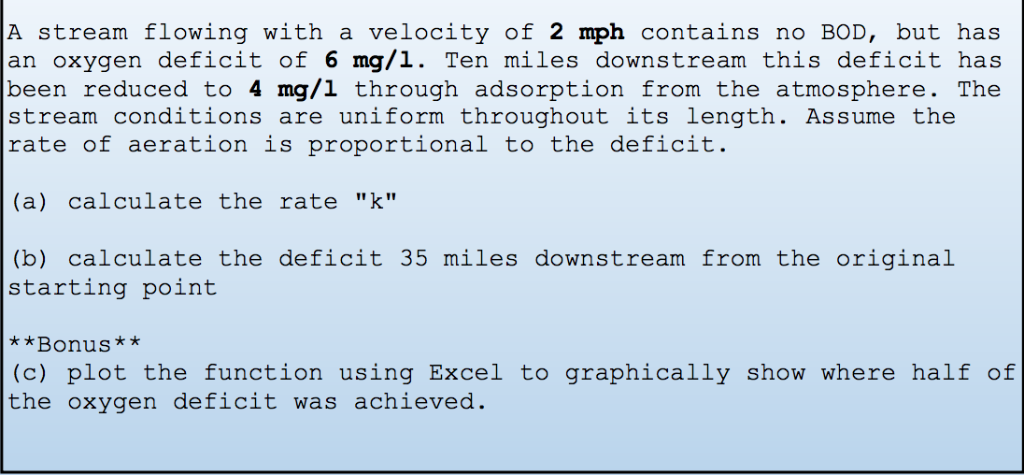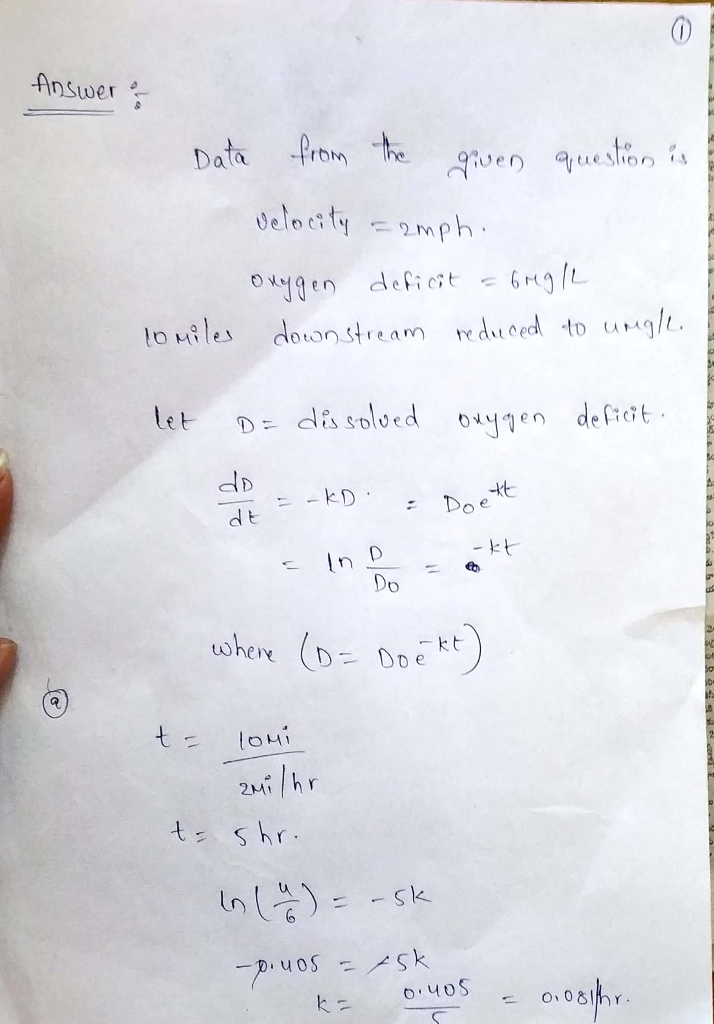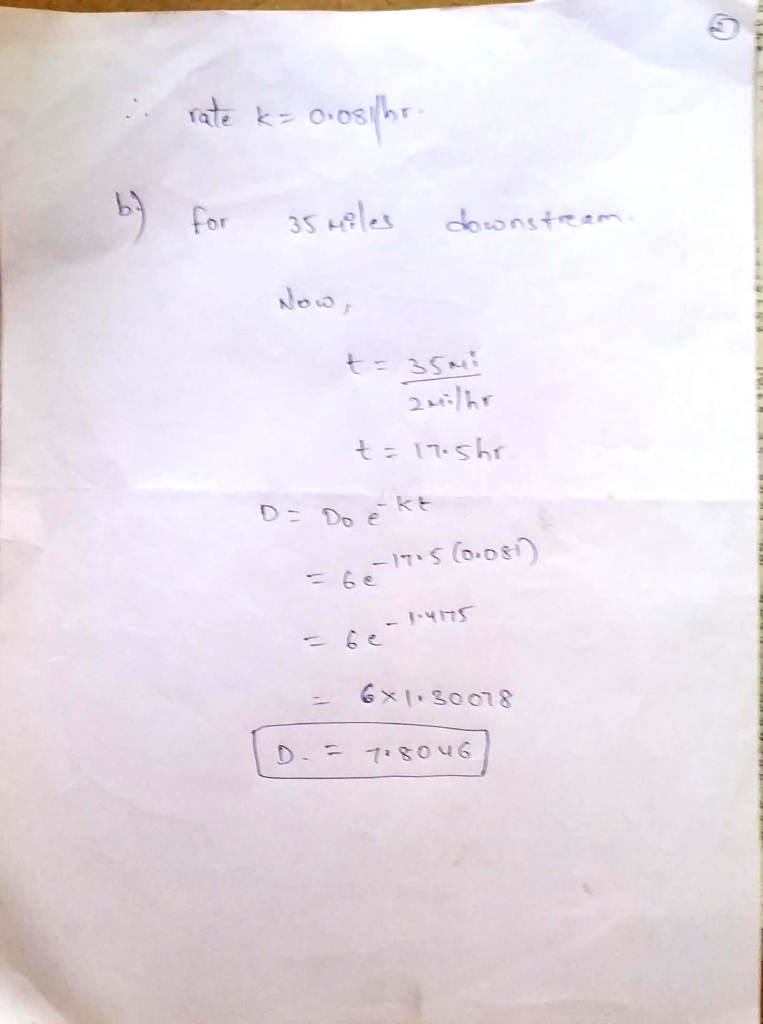# A stream flowing with a velocity of 2 mph contains no BOD, but has an oxygen deficit of 6 mg/1. T...A stream flowing with a velocity of 2 mph contains no BOD, but has an oxygen deficit of 6 mg/1. Ten miles downstream this deficit has been reduced to 4 mg/l through adsorption from the atmosphere. The stream conditions are uniform throughout its length. Assume the rate of aeration is proportional to the deficit. (a) calculate the rate "k" (b) calculate the deficit 35 miles downstream from the original starting point **Bonus (c) plot the function using Excel to graphically show where half of the oxygen deficit was achieved.